Courses

## 20 Questions MCQ Test Quantitative Aptitude for SSC CGL | Test: Quadratic Equations- 2

Description
This mock test of Test: Quadratic Equations- 2 for GMAT helps you for every GMAT entrance exam. This contains 20 Multiple Choice Questions for GMAT Test: Quadratic Equations- 2 (mcq) to study with solutions a complete question bank. The solved questions answers in this Test: Quadratic Equations- 2 quiz give you a good mix of easy questions and tough questions. GMAT students definitely take this Test: Quadratic Equations- 2 exercise for a better result in the exam. You can find other Test: Quadratic Equations- 2 extra questions, long questions & short questions for GMAT on EduRev as well by searching above.
QUESTION: 1

### A number x is multiplied with itself and then added to the product of 4 and x. If the result of these two operations is -4, what is the value of x?

Solution:

Given

• x∗x+4∗x=−4
• x2 + 4x + 4 = 0

To Find: value of x?

Approach

1. As we have a quadratic equation x2+4x+4=0
1. , we will solve this equation to find out the value of x

Working Out

x2+4x+4=0

⇒(x+2)2=0

⇒x=−2

QUESTION: 2

### If x and y are non-zero numbers, what is the value of x/y?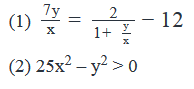Solution:

Steps 1 & 2: Understand Question and Draw Inferences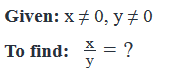Step 3: Analyze Statement 1 independently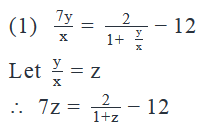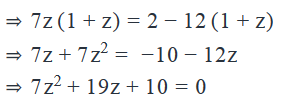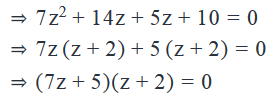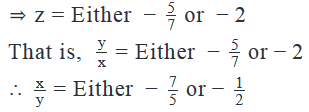Not sufficient to get a unique value of the ratio x/y.

Step 4: Analyze Statement 2 independently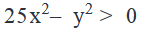Multiplying both sides of the inequality with  will not impact the sign of inequality: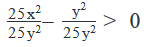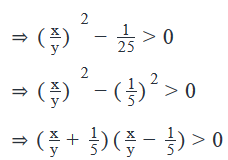From the Wavy Line Method: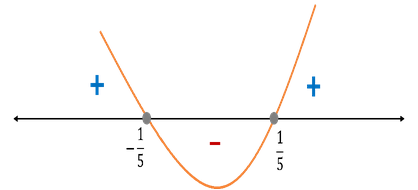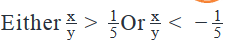Not sufficient to determine the exact value.

Step 5: Analyze Both Statements Together (if needed)

From Statement 1: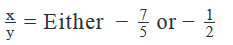From Statement 2: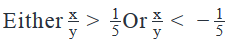Both the values of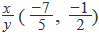obtained from Statement 1 is less than −1/5

Therefore, both these values satisfy Statement 2

So, we are still not able to determine a unique value of the ratio x/y

QUESTION: 3

### If one of the roots of the quadratic equation x2 + bx + 98 = 0 is the average (arithmetic mean) of the  roots of the equation x2 + 28x – 588 = 0, what is the other root of the equation x2 + bx + 98 = 0?

Solution:

Given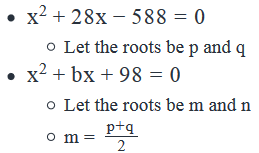To Find: value of n?

Approach

1. We know that the product of roots m and n is equal to c/a
1. So, we can write m*n = 98, i.e. n = 98/m
2. So, for finding n, we need to find m
• We are given that m = (p+q)/2
1. We know that sum of roots of the quadratic equation is −b/a
2. We will use this relation to find the value of p + q and hence the value of m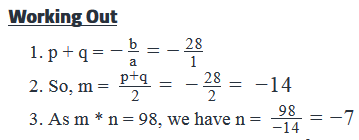Hence, the other root of the equation x2 + bx + 98 = 0 is -7

QUESTION: 4

If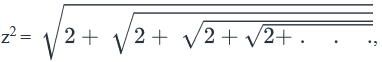where the given expression extends till infinity, which of the following statements must be true ?

I. Two values are possible for z

II. 4 - z2 = 2

III. z8 = 16

Solution:

Given:.

To find: Which of the given 3 statements must be true about z?

Approach:

1. Looking at the 3 statements, we realize that in order to validate them, we’ll need to know the value of z
2. We’re given an expression for z2. Using that expression, we’ll find the value of z

Working Out:

• Finding the value of z
•• Notice that the series of  within  within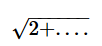is never-ending. So, we can write: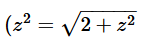To make our calculations easy, let’s replace z2 with another variable, say 'y'

• So, we get: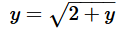• Now, squaring both sides:

• y2 = 2 + y
• y2 – y – 2 = 0
• (y-2)(y+1) = 0
• So, y = 2 or y = -1
• Since y = z2 and perfect squares are never negative, y cannot be -1
• So, y = z2 = 2
• This means, either  z√2   or z-√2
• Checking the validity of the 3 Statements
• Statement I: Two values are possible for z
• As we’ve found above, indeed only 2 values are possible for z
• Therefore, this statement is true
• Statement II: 4 - z2 = 2
• As we calculated above, z2 = 2
• So, 4 – z2 = 4 – 2 = 2
• So, this statement too is true
• Statement III: z8 = 16
• Since z2 = 2, (z2)4 = 24
• So, z8 = 16
• Therefore, this statement is true as well
• Looking at the answer choices, we see that the correct answer is Option D

QUESTION: 5

r and s are the roots of the quadratic equation ax2 + bx + c = 0 where a ≠ 0 & s >0, such that r is 50 percent greater than s. If the product of the roots of the equation is 150, what is the sum of the roots of the equation?

Solution:

Given

• ax2+bx+c=0
• a ≠ 0
• s>0
• Roots of the equation are (r, s)
• r = s + 50% of s = 1.5s
• r * s = 150

To Find:  r + s?

Approach

1. For finding the values of r + s, we need to find the values of r and s
2. We are given two relations between r and s, which are:
a.  r = 1.5s and
b.  r * s = 150
3. As we have 2 equations and 2 variables, we can find the values of r and s and hence the value of r + s.

Working Out

1. Finding values of r and s

r=1.5s……..(1)

r*s = 150……..(2)

Substituting r = 1.5s in (2), we have

1.5s2 = 150

s= 100, i.e. s = 10 or -10

1. If s = 10, r = 15
a. So, r + s = 10 + 15 = 25
2. If s = -10,
1. However, we are given s >0
2. Hence, we can reject this case.
3. So, the value of r + s = 25

Hence the sum of the roots of the equation ax2+bx+c=0

is 25

QUESTION: 6

For a quadratic equation, both the product of the roots and the sum of the roots are prime numbers less than 10. If both the roots are integers, what is the difference between the roots?

Solution:

Given

• Let the roots be p and q, where p, q are integers
• p + q = prime number less than 10
• p + q = {2, 3, 5, 7}
• p*q = prime number less than 10
• So, p*q = {2, 3, 5, 7}
• Since the product of integers p and q is positive, this means that p and q are either both positive or both negative
• But since p + q is positive, this means that p and q cannot both be negative.
• Therefore p, q > 0

To Find:  Value of |p – q|?

• Note: we wrote the expression for difference between the roots as |p-q| and not simply p – q, because at this point, we do not know which of the 2 roots is bigger. By asking about the difference between the 2 roots, the question is asking us to find the value of (Bigger Root – Smaller Root)

Approach

1. To find the value of p – q, we need to find the value of p and q.
2. We know that pq = {2, 3, 5, 7}, i.e. product of two positive integers is prime, Now, the only possible way in which a prime number can be expressed as a product of two positive integers is (Prime number * 1)
3. Using the above information, we can find the possible values of (p, q) = (Prime number, 1), irrespective of the order (that is, irrespective of whether p = Prime Number and q = 1 or vice-versa).
4. Finally, we’ll eliminate those values of (p,q) for which p + q is not prime

Working Out

1. As pq = {2, 3, 5, 7}, possible values of (p, q) can be:

1. (p, q) = (2, 1) or (3, 1) or (5, 1) or (7, 1)

2. Checking if the sum p + q is prime for these possible values of (p,q):

1. If (p, q) = (2, 1), p + q = 3, which is prime
2. If (p, q) = (3, 1), p + q = 4, which is not prime
3. If (p, q) = (5, 1), p + q = 6, which is not prime
4. If (p, q) = (7, 1), p + q = 8, which is not prime

3. Hence, the only possible case where both pq and p + q are prime is when (p, q) = (2, 1), irrespective of the order.

4. So, p – q = 2 – 1 = 1

QUESTION: 7

A quadratic equation ax2 + bx + c = 0 has two integral roots x1 and x2. If the square of the sum of the roots is 6 greater than the sum of the squares of the roots, which of the following could be the value of the ordered set (a, b, c)?

I. (-1, 4, -3)

II. (1, 4, 3)

III. (3, -10√3, 9)

Solution:

Given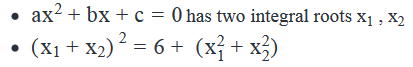To Find: Values of (a, b, c)

Approach

• For finding the values of (a, b, c), we would first need to find the value of x1 , x2
• We will use the relation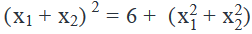• to find out the values of x1 , x2
• Also, we will keep in mind the constraint that x1 , x2 are integers
• Now, we know that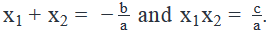• We will use the above relation to find out the possible values of (a, b, c)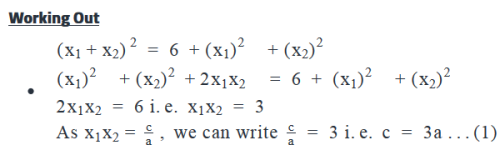As x1 , x2 are integers, the possible cases for (x1 , x2) is either  (3,1) or (-3,-1)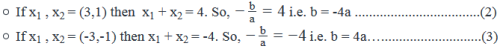•  Using (1), (2) and (3), the values of (a, b, c) can be of the form ( a, 4a, 3a) or (a, -4a, 3a)
• Among the options,
• Option-I (-1, 4, -3) is of the form (a, -4a, 3a) and
• Option- II (1, 4, 3) is of the form (a, 4a, 3a)

Hence, options I and II can be the value of ordered set (a, b, c).

QUESTION: 8

x and y are integers such that xy < 0. If x2 – y2 = 0, what is the value of y?

(1) 2x2 – 21x – 36 = 0

(2) y2 = (-1)n (12y), where n is a positive integer not divisible by 2.

Solution:

Steps 1 & 2: Understand Question and Draw Inferences

• x, y are integers and xy < 0
• x ≠ 0 and y ≠ 0
• x and y are of opposite signs
• x2 – y2 = 0
• (x+y) (x-y) = 0
• Either x = y or x = -y
• As x and y are of opposite signs, the case of x = y can be rejected.
• Therefore, x = -y

To Find: Value of y

Step 3: Analyze Statement 1 independently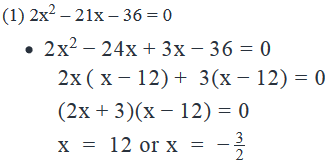• As x is an integer, the case of x=−3/2 is rejected.
• Therefore x = 12
• Thus y = -x = -12

Step 4: Analyze Statement 2 independently

(2) y2 = (-1)n (12y), where n is a positive integer not divisible by 2.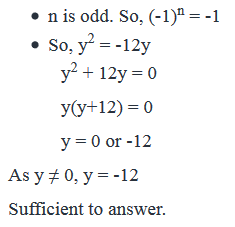Step 5: Analyze Both Statements Together (if needed)

As we have a unique answer from steps 3 and 4, this step is not required.

QUESTION: 9

If p and q are the roots of the quadratic equation ax2 + bx + c = 0 where a ≠ 0, what are the roots of the equation ayx2 + byx + cy = 0 where 0 < y ≤ 1?

Solution:

Given

• ax2+bx+c=0
•  has two roots p and q a ≠ 0, 0 < y ≤ 1

To Find

• Roots of ayx2+byx+cy=0

Approach

1. To find the roots of the equation ayx2+byx+cy=0 , we will do the following steps:
1. Take y common and the get the equation in terms of ax2+bx+c=0
2. As we know the roots of ax2+bx+c=0, we will use this knowledge to find the roots of the equation ayx2+byx+cy=0
•

Working Out

Solving the equation ayx2+byx+cy=0

• ayx2+byx+cy=0
• y(ax2+bx+c)=0

So we have either y = 0 or ax2+bx+c=0

Since we are given that y > 0, y ≠ 0.

Hence ax2+bx+c=0

.As the roots of the equation ax2+bx+c=0  are p and q, the roots of the equation ayx2+byx+cy=0  will also be p and q.

QUESTION: 10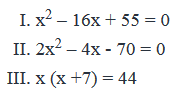Given the three quadratic equations above, which pair of equations has at least one common root?

Solution:

Given

• x2 – 16x + 55 = 0
• 2x2 – 4x - 70 = 0
• x (x +7) = 44

To Find: Which pair of equations has at least one common root?

Approach

1. As we need to find the common roots among the equation, we will solve each quadratic equation to find their roots and then find the pair of equations that have at least one common root.

Working Out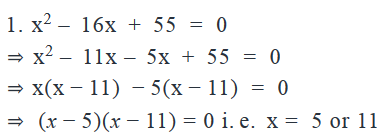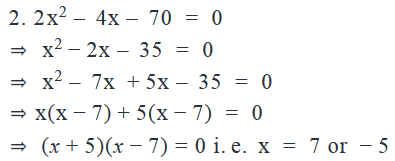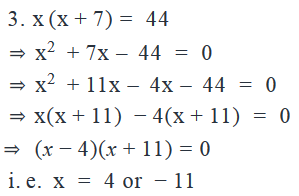So, the roots of Equations I, II and III are (5, 11), (-5, 7) and (-11, 4) respectively.

Hence, none of the equations have even one root in common.

QUESTION: 11

if  2x2 = 24 -x4  and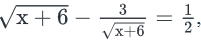which of the following can be the value of x?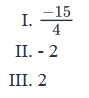Solution:

Given: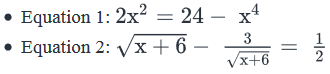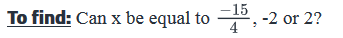Approach:

1. To answer the question, we need to know the possible values of x.
2. So, we’ll first solve Equation 1, then Equation 2 and then find the common roots of both these equations. These will be the possible values of x
3. Then, we’ll evaluate the 3 options.

Working Out:

• Solving Equation I
•  2x2 = 24 -x4
• We can factorize this equation as it is, but if powers like x4 intimidate you, then it’s better to substitute x2 with a new variable, say z. That ways, the equation will look simpler:  2z = 24 – z2
• Rearranging the terms: z2 + 2z – 24 = 0
• z2 + 6z – 4z – 24 = 0
• z(z+6) – 4(z+6) = 0
• (z+6)(z-4) = 0
• This means, z = -6 or 4
• But z = x2. So, x2 = -6 or 4
• Perfect square x2 cannot be negative. Therefore, we can reject the negative root.
• So, x2 = 4
• Therefore, x = +2 or -2
• Thus, Equation I has 2 roots: 2 and -2
• Solving Equation II
•• This equation looks difficult primarily because it involves a square root term,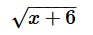. So, for the ease of our calculations, let’s substitute=y
• So, the equation becomes: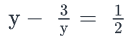• Multiplying both sides with 2y, we get: 2y2 – 6 = y
• Rearranging the terms: 2y2 – y – 6 = 0
• ​​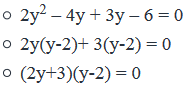• This means, y = -3/2 or y = 2
• Since y =we can write:
•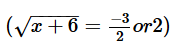• However, since the square root of a number is always positive, the value ofcannot be -3/2 .
• If you’ve any doubt about this, think: When you write z2 = 16 and then take the square root on both sides, you write:  Either z = √16 or z = -√16. Note here that, in the ‘or’ case, you put the minus sign outside the value of √16. This minus sign makes z negative (we get z = -4 in the ‘or’ case) but √16 is always positive (equal to 4).
• Similarly, if we have z2 = x + 6,  then on taking the square root on both sides, we will write: Eitheror
• z= -So, z may be positive or negative butwill always be positive.
• So,=2
• Upon squaring both sides of this equation, we get: x + 6 = 4
• Therefore, x = -2
• Thus, we get a single value of x from Equation II
• Finding the possible values of x
• From Equation I: x = 2 or -2
• From Equation II: x = -2
• So, the value of x that satisfies both equations: x = -2
• Thus, only 1 value of x is possible: {-2}
• Evaluating the 3 options
• Out of the 3 given options, x can only be equal to -2
• Looking at the answer choices, we see that the correct answer is Option B

QUESTION: 12

If x is a negative number, what is the value of x?

(1) 16x2 – 16x – 5 = 0

(2) ||4x+3| - 5| = 3

Solution:

Steps 1 & 2: Understand Question and Draw Inferences

Given: x < 0

To find:  x = ?

Step 3: Analyze Statement 1 independently

(1) 16x2 – 16x – 5 = 0

x2–x−5/16=0

• 2 values of x (roots) will be obtained from this quadratic equation
• Product of these 2 values  = −5/16
• Since the product is negative, one root is positive and the other is negative
• The positive root will be rejected (given: x < 0)

Thus, a unique value of x will be obtained from Statement 1

Statement 1 is sufficient to answer the question.

Step 4: Analyze Statement 2 independently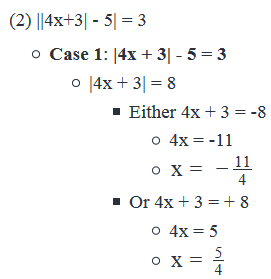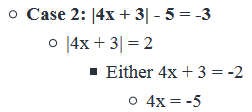x= -5/4 We can reject this value as we know that x < 0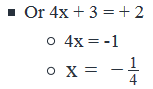Thus, we get 3 possible negative values of x from Statement 2: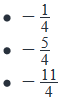Not sufficient to find a unique value of x

Step 5: Analyze Both Statements Together (if needed)

Since we get a unique answer in Step 3, this step is not required

QUESTION: 13

Edward invested five-ninths of his money at an annual rate of 2r% compounded semi-annually, and the remaining money at an annual rate of r% compounded annually. If after one year, Edward’s money had grown by one-thirds, the value of r is equal to which of the following?

Solution:

Given: Let the total money be y

• First Investment:
•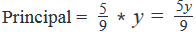• Rate of interest = 2r% p.a. compounded every 6 months = r% per 6-months
• Time = 1 year
• Second Investment:
•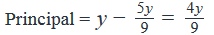• Rate of interest = r% p.a.
• Time = 1 year
• Total Interest earned in 1 year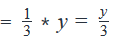To find:  The value of r

Approach

1.  Total interest earned in 1 year = (Interest earned from 1st investment) + (Interest earned from 2nd investment)

i.  In the given time frame of 1 year, the 1st investment will pay interest twice (since this investment pays interest every 6 months). So, the formula for compound interest will be applicable for the 1st investment

ii.  The 2nd investment pays interest after 1 year. Since the given time frame is also 1 year, this investment will yield simple interest

2.  The only unknown in the above equation will be r. So, using this equation, we can find the value of r

Working Out

• Amount of the first investment after 1 year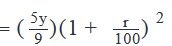• Compound Interest earned from 1st investment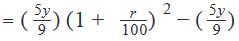1. Calculating the interest earned from the 2nd investment:
1. Simple interest earned from 2nd investment =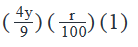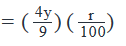2. So, Total interest earned in 1 year =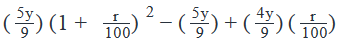1.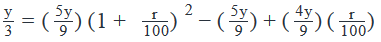2. Multiplying both sides of the above equation with 9/y :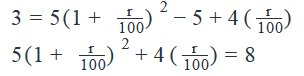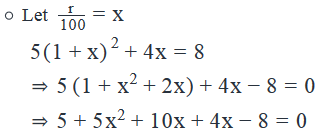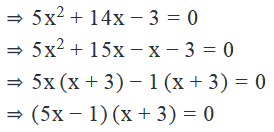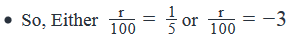• Rejecting the negative value since the money is given to have grown.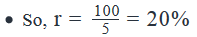Looking at the answer choices, we see that Option C is correct

(Note: You could also have solved this question by framing the first equation in terms of the amount that each investment grows to, as under:

(Total Amount after 1 year) = (Amount that the 1st investment grows to) + (Amount that the 2nd investment grows to)

This equation leads to a similar calculation and the same result as in the solution above /End of Note)

QUESTION: 14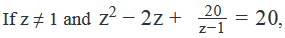then how many negative values can z take ?

Solution: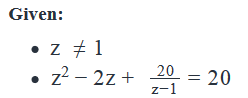To Find: Number of negative values of z

Approach:

• Solve the given equation
• Note that the denominator is z - 1.
• To remove the denominator, we will need to multiply each term of this equation with z - 1.
• This will be time consuming (because we will have to multiply each term with z as well as with -1. Example: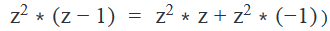and is likely to result in a tedious calculation.
• To simplify this process, we will substitute (z - 1) as x
• Find the value of z
• Count the number of negative values of z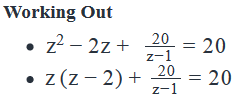Substitute z - 1 = x

Therefore z = x + 1 ; z - 2 =  x -1

Hence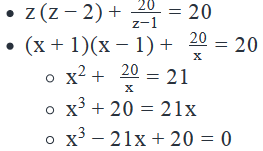This is a cubic equation (involves x3) and you may feel that you do not know how to solve a cubic equation.

However, before giving up, think about how you solve a quadratic equation? By rewriting the given equation into its factors.

Let’s try if the cubic equation above can be similarly written into factors. We’ll find that the middle term, -21x, can be broken down as under: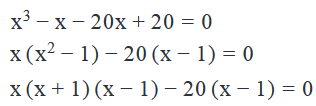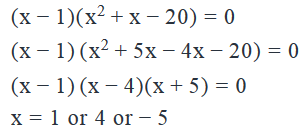That is

• z - 1 = 1 or 4 or -5
• z = 2 or 5 or -4
• Thus, we get three values of z. However, the question asks specifically about the number of negative values of z.
• Among the 3 possible values of z, we see that only one value of z is negative.

QUESTION: 15

If x is equal to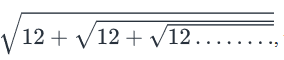, where the given expressions extend to an infinite number of roots, then what is the value of x?

Solution:

Given

•to an infinite terms
• As the value of x is equal to the square root of something, x ≥ 0

To Find:

The value of x

Approach

x=to an infinite terms.

• Since the expression under the root extends infinitely, the expression under the root will be equal to x itself.
• So,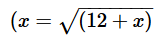0………….(1)
• By solving this equation and applying the constraint that x ≥0, we will find the value of x

Working Out

a.b. Squaring both sides, we have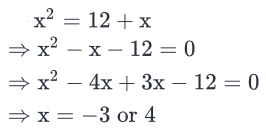As we know that x≥ 0, x = 4

Alternate solution

• Once we have deduced that x will be positive the options -3 and -4 are eliminated
• We know √9 is 3. So the value of √(12+ ……) will  be more than 3. Now we are left with 4 and 12 in the options
• Now √(12 + …..) cannot give us 12..
• So the answer has be 4.
QUESTION: 16

If p and q are the roots of the quadratic equation ax2 + bx + c = 0, where a*b*c ≠ 0, is the product of p and q greater than 0?

(1) |p + q| = |p| + |q|

(2) ac > 0

Solution:

Steps 1 & 2: Understand Question and Draw Inferences

• ax2+bx+c=0
•  where a*b*c ≠ 0
• This means, a ≠ 0, b ≠ 0 and c ≠ 0
• p and q are roots of the equation

To Find: pq > 0?

• Is pq > 0?
• pq > 0, if p and q are of the same signs
• pq < 0, if p and q are of the opposite signs
• Also, Product of roots = pq = c/a
• So, the question becomes: Is c/a>0?
• c/a>0?  , if c and a are of the same signs
• c/a<0  , if c and a are of the opposite signs

So, we need to find the signs of either (p and q) of (c and a).

Step 3: Analyze Statement (1) independently

(1) |p + q| = |p| + |q|

We know, pq = c/a , where a ≠ 0 and c ≠ 0, So p ≠ 0 and q ≠ 0

Now, we know that |x| = x if x ≥ 0 and |x| = -x if x < 0.Since, we have |p| and |q|, following cases are possible:

1. p > 0 and q >0
• As p > 0 and q > 0, we have |p| = p and |q| = q
• So, p + q > 0
• Hence, we can write |p+q| = p +q and |p| +|q| = p + q.
• So, |p+q| = |p| + |q|
• Thus, the equation in statement-1 holds true for p > 0 and q > 0
• In this case pq > 0
2. p > 0 and q < 0
• As p > 0, we have |p| = p and as q < 0, we have |q| = -q
• Now, |p+q| = p + q, if p + q > 0 or –(p+q), if p + q < 0
• Also, |p| +|q| = p - q
• So, for both the values of |p+q|, we have  |p + q| ≠ |p| + |q|
• Thus, the equation in statement-1 does not hold true for p >0 and q < 0
3. p < 0 and q >0
• As p < 0, we have |p| = -p and as q >0, we have |q| = q
• Now, |p+q| = p + q, if p +q ≥ 0 or –(p+q) if p + q < 0
• Also,  |p| + |q| = -p +q
• So, for both the values of |p+q|, we have |p + q| ≠ |p| + |q|
• Thus, the equation in statement-1 does not hold true for p < 0 and q >0
4. p < 0 and q < 0
• As p < 0, we have |p| = -p and as q < 0, we have |q| = -q
• Now, |p+q| = - (p+q) because p + q < 0
• Also,  |p| + |q| = -p – q = - (p+q)
• So, |p+q| = |p| + |q|
• Thus, the equation in statement-1 holds true for p < 0 and q < 0
• In this case pq > 0

So, |p+q| = |p| +|q| is possible only when p, q > 0 or p,q < 0

So in both the cases pq will be greater than 0.

Step 4: Analyze Statement (2) independently

(2) ac > 0

Tells us that a and c have the same signs. Thus c/a>0

and hence pq > 0.

Step 5: Analyze Both Statements Together (if needed)

As we have a unique answer from step 3 and step 4, this step is not required.

Learning

• |a+b| = |a| + |b| if a and b have same sign
• |a+b| < |a| + |b| if a and b have different sign

Alternatively,

• |a-b| = |a| - |b| if a and b have same sign
• |a-b| > |a| -|b| if a and b have different sign
QUESTION: 17

The area of a rectangle is 28 square centimeter. What is the perimeter of the rectangle?

(1) If the length of the rectangle is increased by 10 centimeter and the breadth is decreased by 5 centimeter, the perimeter of the rectangle is eight times the original length of the rectangle.

(2) If the length of the rectangle is increased by 350% and the breadth is increased to 350% of the original breadth, the perimeter of the rectangle is 63 centimeters more than the original perimeter of the rectangle.

Solution:

Steps 1 & 2: Understand Question and Draw Inferences

Given:

Let the length and breadth of rectangle be L and B respectively

• LB = 28…..............................................(1)

To find:  The value of 2(L+B)

• To find perimeter, we need to know the value of L and B.

Step 3: Analyze Statement 1 independently

(1) If the length of the rectangle is increased by 10 centimeter and the breadth is decreased by 5 centimeter, the perimeter of the rectangle is eight times the original length of the rectangle.

• New L = L  +10
• New B = B - 5

So,

• New Perimeter = 2(L+10 + B-5)
• 2(L+10 + B-5) = 8L
• 2L + 2B + 10 = 8L
• 6L – 2B – 10 = 0
• 3L – B – 5 = 0
• B = 3L - 5 . . . . . . . . . . . . . . .. . . . .(2)

Substituting (2) in (1):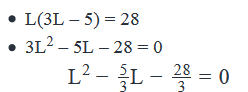• 2 values of L (roots) will be obtained from this quadratic equation

Comparing this with the standard quadratic form : ax2 + bx + c = 0, we get : a = 1 ; b = -5/3 ; c = -28/3

• Product of these 2 values  = (c/a)  −283
• Since the product is negative, one root is positive and the other is negative
• The negative root will be rejected
• L, being the length of a rectangle, cannot be negative
• Thus, a unique value of L is obtained
• Using Equation (2), a unique value of B is also obtained

Hence, Statement 1 alone is sufficient.

Step 4: Analyze Statement 2 independently

• If the length of the rectangle is increased by 350% and the breadth is increased to 350% of the original breadth, the perimeter of the rectangle is 63 centimeters more than the original perimeter of the rectangle
• New L = 4.5L
• New B = 3.5B

So,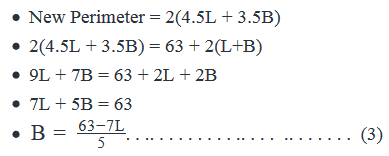Substituting (3) in  (1):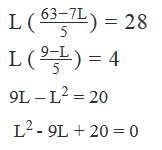• 2 values of L (roots) will be obtained from this quadratic equation

Comparing this with the standard quadratic form : ax2 + bx + c = 0, we get :

a = 1 ; b = - 9 ; c = 20

• Product of these 2 values  = (c/a) =  20
• Since the product is positive, the two roots are either both positive or both negative
• Sum of roots = (-b/a) =  -(-9) = 9
• Since the sum is positive, it means both roots are positive
• Thus, St. 2 leads to 2 values of L
• From Equation (3), 2 values of B will be obtained
• So, 2 values of Perimeter will be obtained.

St. 2 is not sufficient to obtain a unique value of the perimeter.

Step 5: Analyze Both Statements Together (if needed)

Since we get a unique answer in Step 3, this step is not required

QUESTION: 18

What are the roots of the quadratic equation x2 + bx + c = 0 if the roots are distinct and at equal distance from 5 on the number line?

(1) The product of the roots of the equation x2 + bx + c = 0 is 21

(2) x – 7 is a factor of the expression 7x2 + 7bx + 7c

Solution:

Steps 1 & 2: Understand Question and Draw Inferences

• x2+bx+c=0
• Let the distance of the roots of the equation from 5 be d.
• So, the roots will be 5 +d and 5 – d
• Since the 2 roots are distinct, d ≠ 0
• The sum of the roots = (5+d) + (5-d) = 10
• So, −b/a=10
• In the given quadratic equation, a, which denotes the coefficient of x2, is 1.
• So b = -10

To Find: As we have assumed the roots of the equation to be (5 +d) and (5 –d), to find the roots, we need to find the value of d

Step 3: Analyze Statement 1 independently

(1) The product of the roots of the equation x2 + bx + c = 0 is 21

• Product of roots = c/a=21
• Since a = 1 in this equation, we can infer that c = 21
• We need to find the roots of x2−10x+21=0
• Since, we know the quadratic equation, we can find the roots of the equation.

Alternate Method:

• Product of roots = (5+d) (5-d) = 21
• 52−d2=21
• d2 = 25 - 21 = 4
d = 2 or -2
• For d = 2
• Roots = 5+2 = 7 and 5-2 = 3
• For d = -2
• Roots = 5-2 = 3 and 5+2 = 7

So, the roots of the equation are 3 and 7

Step 4: Analyze Statement 2 independently

(2) x – 7 is a factor of the expression 7x2 + 7bx + 7c

• x- 7 is a factor of the expression 7(x2+bx+c)

Since 7 is a constant

• (x-7) is a factor of the expression x2+bx+c
•

Therefore,

• x = 7 is one of the roots of the equation x2+bx+c=0

That is

• 5 + d = 7,
• So, d = 2 and hence the other root is 5 – d = 3. OR
• 5 – d = 7
• So, d = -2 and hence the other root is 5 +(-2) = 3

In both the cases, we get the same values of the roots.

Step 5: Analyze Both Statements Together (if needed)

As we have a unique answer from steps 3 and 4, this step is not required

QUESTION: 19

If x2+4x+p=13  , where p is a constant, what is the product of the roots of this quadratic equation?

(1) -2 is one of the roots of the quadratic equation

(2) x2+4x+p=13  has equal roots

Solution:

Step 1 & Step 2: Understanding the Question statement and Drawing Inferences

Given Info:

• x2+4x+p=13
• Rewriting the above quadratic equation in standard form ax2+bx+c=0
• Subtracting 13 from both sides, we have

⇒ x2+4x+p−13=13−13

⇒ x2+4x+p−13=0

To find:

• We need to find the product of the quadratic equation → x2+4x+p−13=0
• To find the product of the roots:
1.  We need to know the roots or
2. Products of roots of the quadratic equation ax2+bx+c=0 , is given by  c/a
• So product of roots of the above quadratic equation will be (p−13)/1 , as →c=p-13 and a=1
• Now since we do not know the value of p-13, we will not be able to determine the product of roots of the quadratic equation.
• Thus we need to analyse the given statements further to determine the value of p, to be able to calculate the product of roots of the quadratic equation.

Step 3: Analyze statement 1 independently

Statement 1:

• -2 is one of the roots of the quadratic equation
• So, -2 will satisfy the given quadratic equation → x2+4x+p−13=0

⇒ (-2)2 + 4(-2) + p - 13 = 0

⇒ 4 - 8 + p - 13 = 0

⇒ p = 17

• Now since we know the value of p, we will be able to find the value of p-13 and thus will be able to calculate the value of product of quadratic equation.
• Hence statement 1 is sufficient to answer the question.

Step 4: Analyze statement 2 independently

Statement 2:

• Quadratic equation has equal roots
• For equal roots, we will use the relation of sum of roots of the quadratic equation to determine the value of the equal root.
• Sum of roots of the quadratic equation ax2+bx+c=0 , is −b/a
• So sum of roots of the quadratic equation → x2+4x+p−13=0  will be −4/1  , where b=4 and a=1
• Now since both roots are equal and the sum of the roots is coming as -4, both roots will thus be equal to – 2 each.
• Now, the equal root → -2, will satisfy the given quadratic equation x2+4x+p−13=0

⇒(-2)2 + 4(-2) + p - 13 = 0

⇒ p=17

• Now since we know the value of p, we will be able to find the value of p-13 and thus will be able to calculate the value of product of quadratic equation.
• Hence statement 2 is sufficient to answer the question

Step 5: Analyze the two statements together

• Since from statement 1 and statement 2, we are able to arrive at a unique answer, combining and analysing statements together is not required.

Hence the correct answer is option D

QUESTION: 20

x2 + bx + 72 = 0 has two distinct integer roots; how many values are possible for 'b'?

Solution:

In quadratic equations of the form ax2 + bx + c = 0. - b/a  represents the sum of the roots of the quadratic equation and c/a represents the product of the roots of the quadratic equation.

In the equation given a = 1, b = b and c = 72
So, the product of roots of the quadratic equation = 72/1 = 72
And the sum of roots of this quadratic equation = -b/1 = -b
We have been asked to find the number of values that 'b' can take.
If we list all possible combinations for the roots of the quadratic equation, we can find out the number of values the sum of the roots of the quadratic equation can take.
Consequently, we will be able to find the number of values that 'b' can take.

The question states that the roots are integers.
If the roots are r1 and r2, then r1 * r2 = 72, where both r1 and r2 are integers.
Possible combinations of integers whose product equal 72 are : (1, 72), (2, 36), (3, 24), (4, 18), (6, 12) and (8, 9) where both r1 and r2 are positive. 6 combinations.

For each of these combinations, both r1 and r2 could be negative and their product will still be 72.
i.e., r1 and r2 can take the following values too : (-1, -72), (-2, -36), (-3, -24), (-4, -18), (-6, -12) and (-8, -9). 6 combinations.

Therefore, 12 combinations are possible where the product of r1 and r2 is 72.
Hence, 'b' will take 12 possible values.